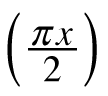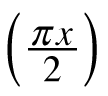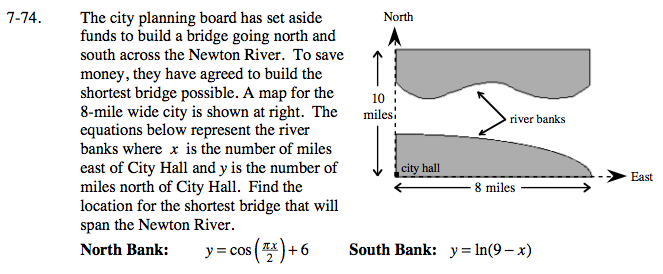### Home > CALC > Chapter 7 > Lesson 7.2.2 > Problem7-74

7-74.

The city planning board has set aside funds to build a bridge going north and south across the Newton River. To save money, they have agreed to build the shortest bridge possible. A map for the 8-mile wide city is shown below. The equations below represent the river banks where x is the number of miles east of City Hall and y is the number of miles north of City Hall. Find the location for the shortest bridge that will span the Newton River. Homework Help ✎North Bank: y = cos+ 6
South Bank: y = ln(9 − x)At any give value of x, the distance between the North and South Banks is:

$y=\left ( \text{cos}\left ( \frac{\pi (x)}{2}\right )+6 \right )-(\text{ln}(9-x)) .$

Optimize.